# Squaring the Circle

Squaring the circle is the age old problem of constructing a square with the same area(or perimeter) as the circle. Greek mathematicians attempted to solve this ancient riddle using a ruler and compass only. Due to the transcendental nature of π we can only approximate this geometry.  Earth, Moon, the Great Pyramid, and Stonehenge all encode this great philosophical quandary.Stonehenge encodes the squared circle through its bluestones, named so because when it rains they turn blue.  If we draw a square around this circle of stones it will have the same perimeter as the sarsen ring of stones on the very outside.  The ancients preserved esoteric gnosis in their sacred buildings as a way to ensure that the information would never be lost or occulted by the greed of mortal men.

“ A tradition which has been credited by many learned men over the centuries is that the Ancients encoded their knowledge of the world in the dimensions of their sacred monuments.”
– John Michell

The Great Pyramid’s height is in relationship to its base sides as a circle’s radius is to its circumference, and thus it ‘squares the circle’.  Put another way, the perimeter of the base equals the circumference of a circle whose radius is equal to the height of the pyramid.  This is only achieved due to the slope angle being 51 degrees and 51 minutes. (or 51.84 degrees since there are 60 arc minutes in 1 degree)  I noticed that in the six days it supposedly took to create heaven and earth, there are exactly 518,400 seconds, which resonates with the decad (the decimal system), and the slope angle.  And just recently my friend Dayne Herndon pointed out the fact that 5184 also resonates with the canonical value for the Precession of the Equinoxes of 25,920 years. As 25,920 x 2 = 51,840

What else could the Great Pyramid possibly encode?  How about the Prime Meridian as suggested by Carl Munck?  This is a future post but for now check out The Code.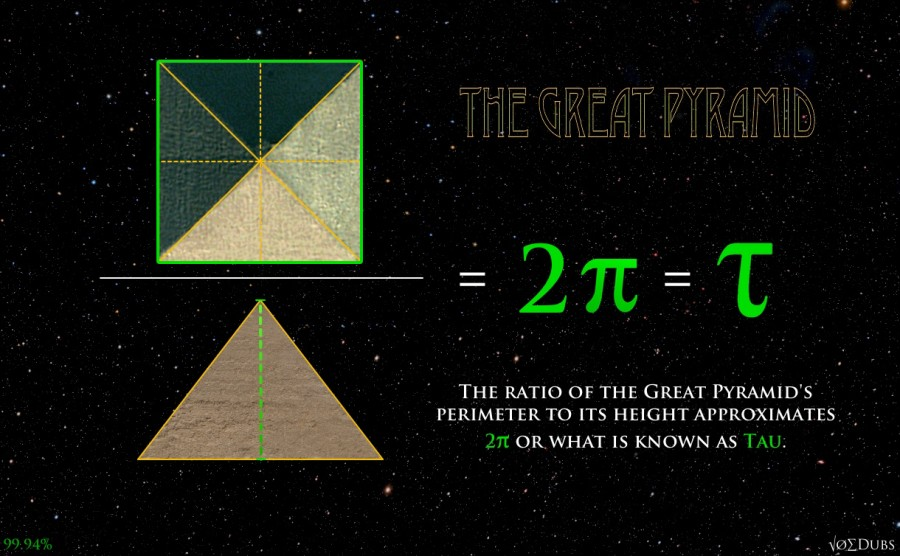“Squaring the circle” is the alchemical process of transferring an airy concept from the mental plane to the physical dimension so that objective conception and birth become a demonstrative reality. – Dr. John Munford

The Earth-Moon relationship and the Great Pyramid of Giza both encode the secret to the mystery.  Both of these correlations are well over 99.9% accurate. This is what Leonardo Da Vinci’s “Vitruvian Man” is all about.

Based on measurements of Leonardo da Vinci’s Vitruvian Man we can see that the sizes of the square and circle aren’t quite the correct size to actually square the area of the circle. (in red) But we can also square the circle with equal perimeters (in blue).  Vitruvius’ square (in yellow) is almost exactly halfway between ‘squaring the circle’ with equal area, and ‘squaring the circle’ with equal perimeters. It’s slightly closer to the latter.

The square that Da Vinci used is a consolidation of two distinct solutions to ‘squaring the circle’, or obtaining the unobtainable. Did Leonardo encode the solution of this ancient philosophical mystery by suggesting that man is the mean between two transcendental impossibilities?

“The workings of the human body are an analogy of the workings of our universe” – Leonardo da Vinci

Squaring the Circle Methods

Equal Areas:
Square with area of 4 has width of 2
Circle with area of 4 has width of 2.256758..

9/8 = 1.125
2.256 / 2 = 1.128379
1.125 / 1.128379 = .9970
A 9:8 ratio squares the area of the circle to 99.70% accuracy
In music theory, a 9 to 8 ratio is the whole step, otherwise known as the whole tone, or major second.

Equal Perimeters:
Square with perimeter of 4 has width of 1
Circle with circumference of 4 has width of 1.2732396..
Interestingly, our Moon takes 27.32166 days to make 1 revolution about Earth.
(A 99.998% accurate correlation according to NASA)

14/11 = 1.27272727…
1.2732396 / 1 = 1.2732396
1.272727 / 1.2732396 = .9996
A 14:11 ratio squares the circle with equal perimeters to 99.96% accuracy

Earth and Moon solve the first riddle to a very high degree of accuracy.  Depending on which measures you use, equatorial, polar and mean diameters, it is at least 99.9% accurate.

Square the Circle/ Earth-Moon Correlations:
(NASA 2014 measurements in miles)

Earth-Moon radii / Earth’s radius = Square the Circle with equal perimeters

5043.175 / 3963.17 = 1.27251039
1.27251039 / 1.2732396 = 99.943% accuracy

5028.63 / 3949.93 = 1.27309
1.27309 / 1.2732396 is 99.988% accuracy

5038.7 / 3958.75 = 1.27280
99.965% accuracy

As you can see you don’t have to cherry pick measurements to achieve a very high degree of accuracy.  Earth is a living and breathing organism.  Its measurements change over time so this could never be 100% accurate.

“The circle is a symbol of spirit, of heaven, of the unmanifest, the immeasurable and the infinite, while the square is the symbol of the material, the Earth, the measurable and the finite,” …the symbolic essence of the problem was the reconciliation of seemingly opposing principles, and the resolution of dualities – “a sacred, cosmological act.” -Daniel Pinchbeck

The Earth and Moon are the perfect size to solve the riddle. This only works because the Moon is huge.  It’s larger than any other of the solar system in proportion to its planet. Some say we have a double planet system.

“The squaring of the circle is a stage on the way to the unconscious, a point of transition leading to a goal lying as yet unformulated beyond it. It is one of those paths to the centre.” – Carl G. Jung

Bert Janssen found the squaring of the circle encoded into certain crop circles.  His website is definitely worth checking out.The crop circle that came down on 2 August 1996 next to Liddington Castle. Nicknamed: the Sun and Moon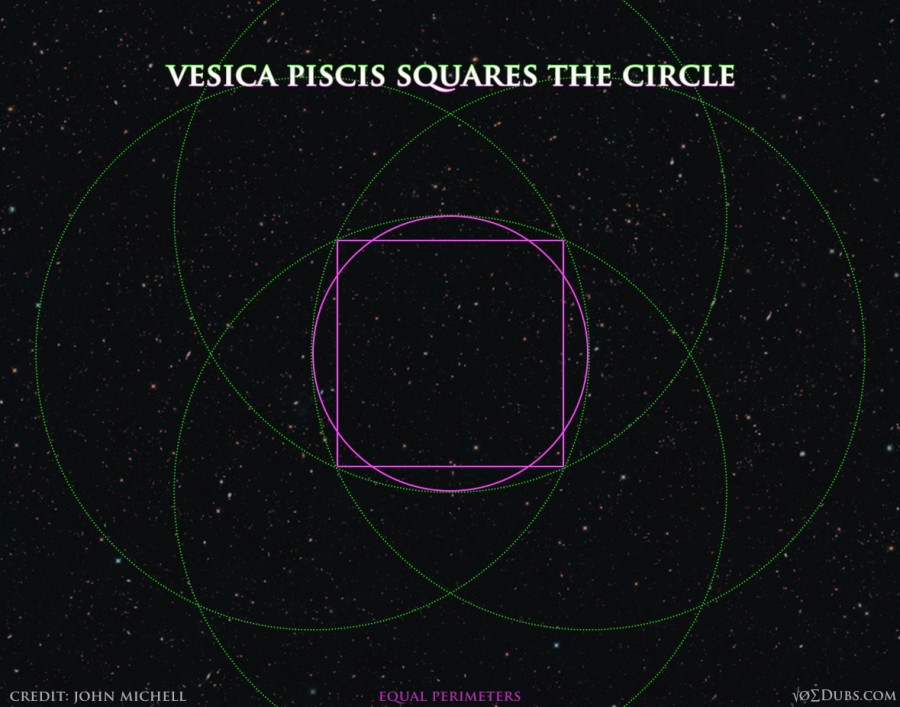The Vesica Piscis Squares the Circle (these two have equal perimeters)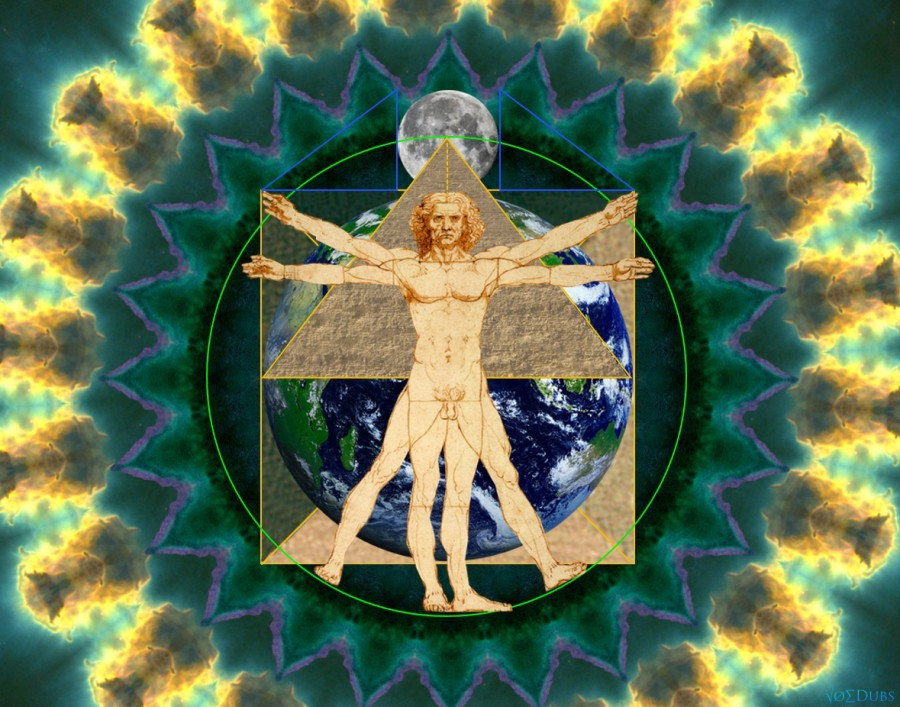The alchemical marriage between Earth and Moon reveal profound geometrical symbols we use to understand our reality.  These relationships are a hint into the mystery of our existence.  Perhaps we are more than mere coincidence and product of time and chance.  The Divine Universal Architect may have hid these clues in plain sight for all to see and cherish, however it is only the few who possess such esoteric knowledge that have the eyes to see these Secrets in Plain Sight.The big circle has about the same circumference as the perimeter of the square

An exercise in contemplative geometry from Robert Lawlor’s Sacred Geometry, 1982.

“There are a number of diagrams in the literature of Sacred Geometry all related to the single idea known as ‘Squaring of the Circle’. This is a practice which seeks, with only the usual compass and straight-edge, to construct a square which is virtually equal in perimeter to the circumference of a given circle, or which is virtually equal in area to the area of a given circle. Because the circle is an incommensurable figure based on π, it is impossible to draw a square more than approximately equal to it. Nevertheless the Squaring of the Circle is of great importance to the geometer-cosmologist because for him the circle represents pure, unmanifest spirit-space, while the square represents the manifest and comprehensible world. When a near equality is drawn between the circle and the square, the infinite is able to express its dimensions or qualities through the finite” (p74).

The derivation begins with an initial circle (within the square) of radius unity. Along its horizontal diameter are drawn two tangent circles, each with radius one half. Observe that the total circumference of the smaller circles equals the circumference of the initial circle, but the total area of the smaller circles is one half that of the initial circle: “One has become Two” (p73), an image of the primary duality, of yin-yang.

Next are drawn two arcs from the ends of the initial circle’s vertical diameter with radius tangent to the far sides of the smaller circles. This radius is φ, the golden ratio, dividing the vertical radius of the initial circle into the golden section of lengths 1/φ and 1/φ2. The two arcs meet to create a vesica that encloses the primary duality — the mouth of Ra, the Word, the vibrating string.

Around the initial circle is drawn a tangent square, with side 2, perimeter 8; and, finally, a large circle is drawn with diameter equal to the width of the vesica, 2√φ, giving a circumference of 2π√φ = 7.993, approximately equal to 8. The circle is squared.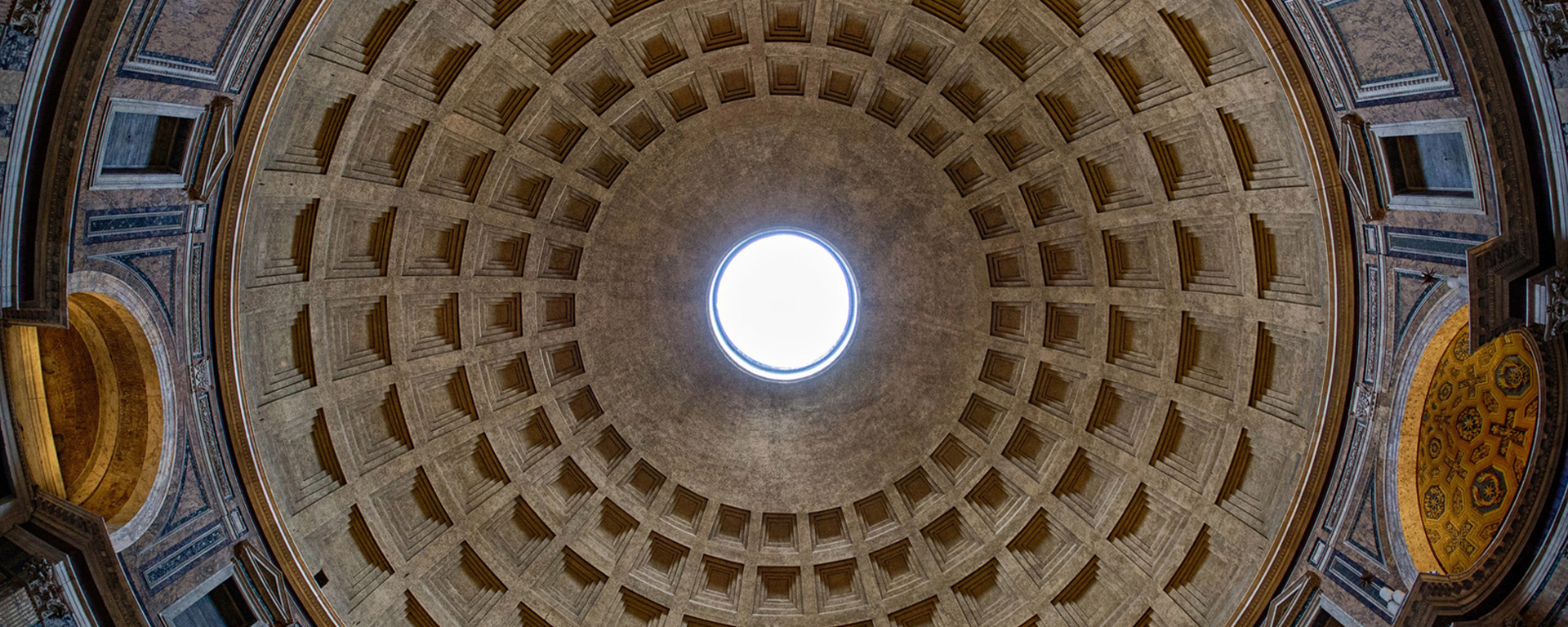“From the domed Pantheon of ancient Rome, if not before, architects have fashioned sacred dwellings after conceptions of the universe, utilizing circle and square geometries to depict spirit and matter united. Circular domes evoke the spherical cosmos and the descent of heavenly spirit to the material plane. Squares and cubes delineate the spatial directions of our physical world and portray the lifting up of material perfection to the divine.

Constructing these basic figures is elementary. The circle results when a cord is made to revolve around a post. The right angle of a square appears in a 3:4:5 triangle, easily made from a string of twelve equally spaced knots.1 But “squaring the circle”—drawing circles and squares of equal areas or perimeters by means of a compass or rule—has eluded geometers from early times.2 The problem cannot be solved with absolute precision, for circles are measured by the incommensurable value pi (S = 3.1415927…), which cannot be accurately expressed in finite whole numbers by which we measure squares.3 At the symbolic level, however, the quest to obtain circles and squares of equal measure is equivalent to seeking the union of transcendent and finite qualities, or the marriage of heaven and earth. Various pursuits draw from the properties of music, geometry and even astronomical measures and distances.”

-RACHEL FLETCHER – Squaring the Circle: Marriage of Heaven and Earth

Squaring the circle with Earth and Moon

The 2732 Moon Mystery

Unknown Squared Circle: More than meets the Eye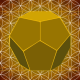## Joe Dubs

I write about philosophy, geometry, health, politics and other stuff that interests me.

1.•Thx Panagiotis, This looks very interesting indeed. 🙂

2.Robert Aguirre

Epogdoon!!!

3.preston

Can you send me a link on how to draw the vesica pisces with the square and circle in the middle?? I cannot seem to figure out how to draw that…the center point of the circles does not fall on the outer circumference like when you draw the flower of life….How are you creating the pattern? how are you connecting the 4 green circles without doing any freehand ?

I would really appreciate a response!!

•I recommend checking out SGD to learn how to construct these types of drawings.